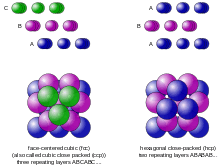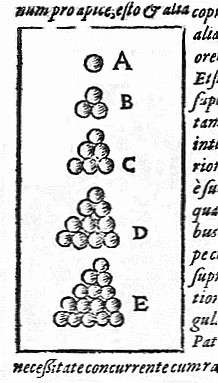# Kepler conjecture

The Kepler conjecture, named after the 17th-century mathematician and astronomer Johannes Kepler, is a mathematical conjecture about sphere packing in three-dimensional Euclidean space. It says that no arrangement of equally sized spheres filling space has a greater average density than that of the cubic close packing (face-centered cubic) and hexagonal close packing arrangements. The density of these arrangements is around 74.05%.

In 1998 Thomas Hales, following an approach suggested by Fejes Tóth (1953), announced that he had a proof of the Kepler conjecture. Hales' proof is a proof by exhaustion involving the checking of many individual cases using complex computer calculations. Referees have said that they are "99% certain" of the correctness of Hales' proof, and now Kepler conjecture is accepted as a theorem. In 2014, the Flyspeck project team, headed by Hales, announced the completion of a formal proof of the Kepler conjecture using a combination of the Isabelle and HOL Light proof assistants.

## BackgroundDiagrams of cubic close packing (left) and hexagonal close packing (right).

Imagine filling a large container with small equal-sized spheres. The density of the arrangement is equal to the collective volume of the spheres divided by the volume of the container. To maximize the number of spheres in the container means to create an arrangement with the highest possible density, so that the spheres are packed together as closely as possible.

Experiment shows that dropping the spheres in randomly will achieve a density of around 65%. However, a higher density can be achieved by carefully arranging the spheres as follows. Start with a layer of spheres in a hexagonal lattice, then put the next layer of spheres in the lowest points you can find above the first layer, and so on. At each step there are two choices of where to put the next layer, so this natural method of stacking the spheres creates an uncountably infinite number of equally dense packings, the best known of which are called cubic close packing and hexagonal close packing. Each of these arrangements has an average density ofThe Kepler conjecture says that this is the best that can be doneno other arrangement of spheres has a higher average density.

## OriginsOne of the diagrams from Strena Seu de Nive Sexangula, illustrating the Kepler conjecture

The conjecture was first stated by Johannes Kepler (1611) in his paper 'On the six-cornered snowflake'. He had started to study arrangements of spheres as a result of his correspondence with the English mathematician and astronomer Thomas Harriot in 1606. Harriot was a friend and assistant of Sir Walter Raleigh, who had set Harriot the problem of determining how best to stack cannonballs on the decks of his ships. Harriot published a study of various stacking patterns in 1591, and went on to develop an early version of atomic theory.

## Nineteenth century

Kepler did not have a proof of the conjecture, and the next step was taken by Carl Friedrich Gauss (1831), who proved that the Kepler conjecture is true if the spheres have to be arranged in a regular lattice.

This meant that any packing arrangement that disproved the Kepler conjecture would have to be an irregular one. But eliminating all possible irregular arrangements is very difficult, and this is what made the Kepler conjecture so hard to prove. In fact, there are irregular arrangements that are denser than the cubic close packing arrangement over a small enough volume, but any attempt to extend these arrangements to fill a larger volume always reduces their density.

After Gauss, no further progress was made towards proving the Kepler conjecture in the nineteenth century. In 1900 David Hilbert included it in his list of twenty three unsolved problems of mathematicsit forms part of Hilbert's eighteenth problem.

## Twentieth century

The next step toward a solution was taken by László Fejes Tóth. Fejes Tóth (1953) showed that the problem of determining the maximum density of all arrangements (regular and irregular) could be reduced to a finite (but very large) number of calculations. This meant that a proof by exhaustion was, in principle, possible. As Fejes Tóth realised, a fast enough computer could turn this theoretical result into a practical approach to the problem.

Meanwhile, attempts were made to find an upper bound for the maximum density of any possible arrangement of spheres. English mathematician Claude Ambrose Rogers (see Rogers (1958)) established an upper bound value of about 78%, and subsequent efforts by other mathematicians reduced this value slightly, but this was still much larger than the cubic close packing density of about 74%.

In 1990, Wu-Yi Hsiang claimed to have proven the Kepler conjecture. The proof was praised by Encyclopædia Britannica and Science and Hsiang was also honored at joint meetings of AMS-MAA. Wu-Yi Hsiang (1993, 2001) claimed to prove the Kepler conjecture using geometric methods. However Gábor Fejes Tóth (the son of László Fejes Tóth) stated in his review of the paper "As far as details are concerned, my opinion is that many of the key statements have no acceptable proofs." Hales (1994) gave a detailed criticism of Hsiang's work, to which Hsiang (1995) responded. The current consensus is that Hsiang's proof is incomplete.

## Hales' proof

Following the approach suggested by Fejes Tóth (1953), Thomas Hales, then at the University of Michigan, determined that the maximum density of all arrangements could be found by minimizing a function with 150 variables. In 1992, assisted by his graduate student Samuel Ferguson, he embarked on a research program to systematically apply linear programming methods to find a lower bound on the value of this function for each one of a set of over 5,000 different configurations of spheres. If a lower bound (for the function value) could be found for every one of these configurations that was greater than the value of the function for the cubic close packing arrangement, then the Kepler conjecture would be proved. To find lower bounds for all cases involved solving around 100,000 linear programming problems.

When presenting the progress of his project in 1996, Hales said that the end was in sight, but it might take "a year or two" to complete. In August 1998 Hales announced that the proof was complete. At that stage it consisted of 250 pages of notes and 3 gigabytes of computer programs, data and results.

Despite the unusual nature of the proof, the editors of the Annals of Mathematics agreed to publish it, provided it was accepted by a panel of twelve referees. In 2003, after four years of work, the head of the referee's panel, Gábor Fejes Tóth, reported that the panel were "99% certain" of the correctness of the proof, but they could not certify the correctness of all of the computer calculations.

Hales (2005) published a 100-page paper describing the non-computer part of his proof in detail. Hales & Ferguson (2006) and several subsequent papers described the computational portions. Hales and Ferguson received the Fulkerson Prize for outstanding papers in the area of discrete mathematics for 2009.

### A formal proof

In January 2003, Hales announced the start of a collaborative project to produce a complete formal proof of the Kepler conjecture. The aim was to remove any remaining uncertainty about the validity of the proof by creating a formal proof that can be verified by automated proof checking software such as HOL Light and Isabelle. This project is called Flyspeck – the F, P and K standing for Formal Proof of Kepler. Hales estimated that producing a complete formal proof would take around 20 years of work. The project was announced completed on August 10, 2014. In January 2015 Hales and 21 collaborators published "A formal proof of the Kepler conjecture".

## Related problems

Thue's theorem
The regular hexagonal packing is the densest sphere packing in the plane. (1890)
The 2-dimensional analog of the Kepler conjecture; the proof is elementary. Henk and Ziegler attribute this result to Lagrange, in 1773 (see references, pag. 770).The density is π12.
The hexagonal honeycomb conjecture
The most efficient partition of the plane into equal areas is the regular hexagonal tiling. Hales' proof (1999).
Related to Thue's theorem.
Dodecahedral conjecture
The volume of the Voronoi polyhedron of a sphere in a packing of equal spheres is at least the volume of a regular dodecahedron with inradius 1. McLaughlin's proof, for which he received the 1999 Morgan Prize.
A related problem, whose proof uses similar techniques to Hales' proof of the Kepler conjecture. Conjecture by L. Fejes Tóth in the 1950s.
The Kelvin problem
What is the most efficient foam in 3 dimensions? This was conjectured to be solved by the Kelvin structure, and this was widely believed for over 100 years, until disproved by the discovery of the Weaire–Phelan structure. The surprising discovery of the Weaire–Phelan structure and disproof of the Kelvin conjecture is one reason for the caution in accepting Hales' proof of the Kepler conjecture.
Sphere packing in higher dimensions
In 2016, Maryna Viazovska announced proofs of the optimal sphere packings in dimensions 8 and 24. However, the optimal sphere packing question in dimensions other than 1, 2, 3, 8, and 24 is still open.
Ulam's packing conjecture
It is unknown whether there is a convex solid whose optimal packing density is lower than that of the sphere.